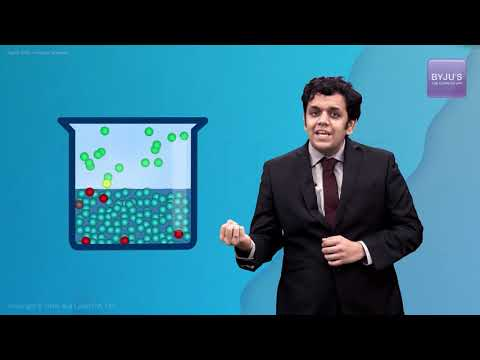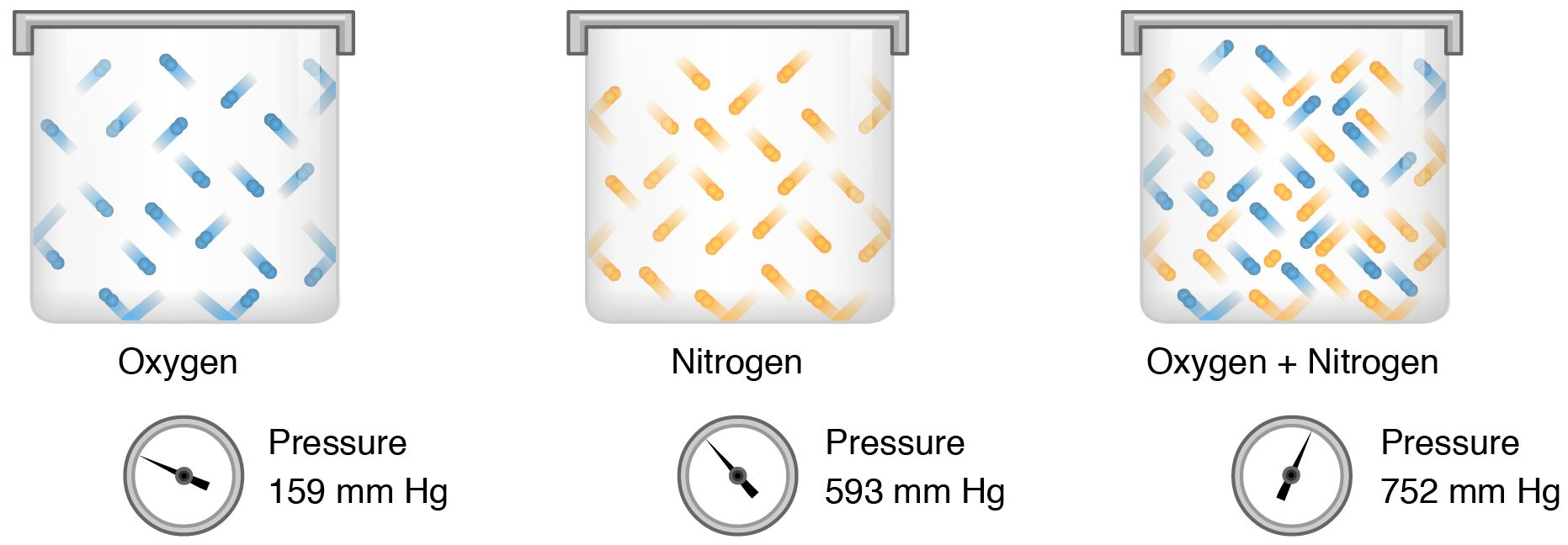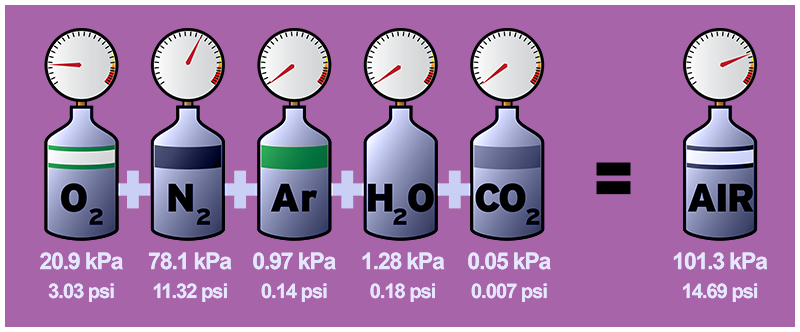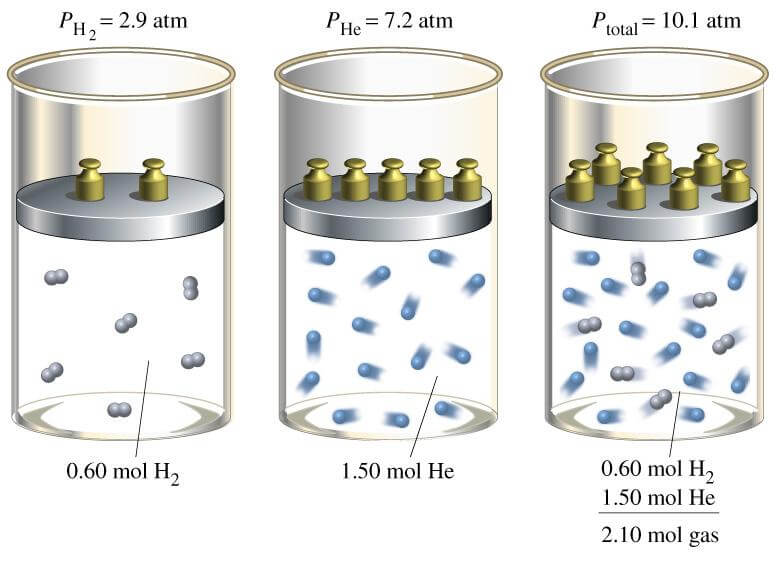Defend yourself better by mastering the science of immunity and vaccines. Secure your free spot, now! Defend yourself better by mastering the science of immunity and vaccines. Secure your free spot, now!

# Partial Pressure

## What is Partial Pressure?

Partial Pressure is defined as if a container filled with more than one gas, each gas exerts pressure. The pressure of anyone gas within the container is called its partial pressure

## Expression of Partial Pressure

The pressure that is exerted by one among the mixture of gases if it occupies the same volume on its own is known as Partial pressure. Every gas exerts certain pressure in a mixture. The total pressure of a mixture of an ideal gas is the sum of partial pressures of individual gases in the mixture, based on the following equation:

$$\begin{array}{l}\frac{V_{x}}{V_{tot}}=\frac{p_{x}}{p_{tot}}=\frac{n_{x}}{n_{tot}}\end{array}$$

• Vx denotes the partial pressure of the particular gas.
• Px indicates the partial pressure of the gas x.
• Vtot denotes the total volume of the mixture.
• Nx indicates the amount of gaseous substance.
• Ptot denotes the total pressure of the mixture.
• Ntot is the total amount of substance in a mixture.Partial Pressure

#### Applying Concepts – Dalton’s law of partial pressure#### States of matter – Dalton law of partial pressure#### Vapour Pressure## Ideal Gases and Partial Pressure

Ideal gas behavior allows gas mixtures to be specified simply. In particular, the ideal gas law holds for each component of the mixture separately. Each component exerts its own pressure referred to as its partial pressure.

Partial pressure is the measure of the thermodynamic activity of gas molecules. The gasses diffuse and react based on their partial pressures and not concentrations in a gaseous mixture.## Dalton’s Law of Partial Pressure

According to Dalton’s law of partial pressures, the total pressure exerted by the mixture of gases is the sum of the partial pressure of every existing individual gas, and every gas is assumed to be an Ideal gas.

Ptotal = P1 + P2 + P3

Where P1, P2, P3 are the partial pressures of gas 1, gas 2, and gas 3. Since every gas has an independent behavior, the ideal gas law is used to find the pressure of that gas if its number of moles, the volume of container and temperature is known.The equality arises because the molecules are so wide apart that there is minimal interaction in an ideal gas. For example, a mixture of an ideal gas that consists of Nitrogen, hydrogen, and ammonia.

P = PN2 + PH2 + PNH3

Where,Q1

### What is partial pressure? Give examples.

The mole fraction of the gas in the mixture determines the partial pressures, and there are no precise values for the gases. For example, if a mixture contains 1 mole gas A and 2 moles gas B and the overall pressure is 3 atm.

Q2

### What is partial pressure and why is it important?

Partial pressure is the force which a gas exerts. The sum of the partial pressures in a mixture of all the gases equals the overall pressure. Partial friction is of paramount significance when forecasting gas flow. Recall that gases in two regions that are connected tend to equalize their pressure.

Q3

### What is the unit for partial pressure?

Partial pressures can be cited in any standard unit of pressure. Popular examples are Pascals (Pa) or atmospheres (atm). Pascals are identical with N m-2 (newtons per square metre). A homogeneous equilibrium is one in which everything is present at the same time in the equilibrium combination.

Q4

### What does partial pressure depend on?

In a mixture, the partial pressure of each gas is proportional to its fraction of the mole. The pressure exerted by each gas (its partial pressure) in a gas mixture is independent of the pressure exerted by all the other gases present.

Q5

### What is partial pressure gas?

Each constituent gas in a mixture of gases has a partial pressure which is the notional pressure of that constituent gas if it alone occupied the whole volume of the original mixture at the same temperature. The overall pressure of an ideal gas mix is the sum of the gases’ partial pressures in the mixture.

To know more about partial pressure of oxygen and how to calculate it and more, register with BYJU’S and download our app.

Test your knowledge on partial pressure